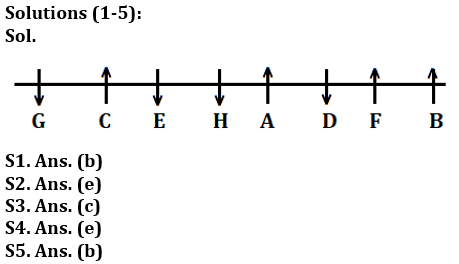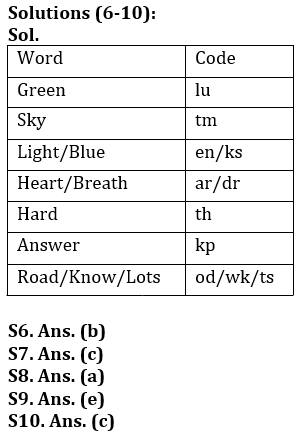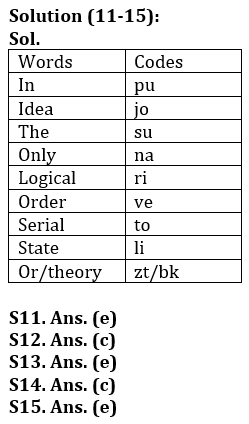Latest Banking jobs   »

# Reasoning Ability Quiz For IDBI AM/ Bank of India PO 2023 -1st March

Directions (1-5): Study the information carefully and answer the questions given below.
Eight persons A, B, C, D, E, F, G and H are sitting in a linear row. Some of them face north and some of them face south. Not more than two persons sit adjacent face same direction.
C sits immediate right of E. G sits one of the extreme ends of the row. Two persons sit between G and H. F sits third to the left of H. immediate neighbor of H face opposite direction to each other. Three persons sit between F and E. C face north direction. A and D sits immediate neighbor to each other. Only one person sits between D and B. F face north direction. The persons sit at extreme ends of the row face opposite direction to each other. G faces same direction of H.

Q1. Four of the following five are alike in certain way based from a group, find the one who does not belong to that group?
(a) G
(b) F
(c) E
(d) H
(e) D

Q2. Who among the following sits immediate left of A?
(a) E
(b) The one who sits immediate left of C
(c) D
(d) The one who sits second to left of D
(e) None of these

Q3. How many persons sit between D and G?
(a) One
(b) Two
(c) More than three
(d) Three
(e) None

Q4. How many persons face south direction?
(a) Two
(b) Five
(c) Three
(d) One
(e) Four

Q5. Which of the following pair sit at extreme end of the row?
(a) D, F
(b) B, G
(c) C, H
(d) D, C
(e) None of these

Directions (6-10): Study the following information carefully and answer the questions given below:
In a certain code language:
‘Light Green Blue Sky’ is written as ‘en lu ks tm’,
‘Heart Sky Breath Hard’ is written as ‘tm ar th dr’,
‘Hard light Blue Answer’ is written as ‘th en kp ks’

Q6. What is the code for ‘Green’ in the given code language?
(a) En
(b) Lu
(c) Ks
(d) Tm
(e) None of these

Q7. What may be the code for ‘light Heart Sky’ in the given code language?
(a) en th tm
(b) lu ks dr
(c) en ar tm
(d) tm en ks
(e) None of these

Q8. What is the code for ‘know lots Road Hard’ in the given code language?
(a) od wk ts th
(b) od en wk ts
(c) kp dr tm od
(d) wk ts od ks
(e) None of these

Q9. What is the code for ‘Sky’ in the given code language?
(a) wk
(b) kp
(c) od
(d) ts
(e) None of these

Q10. What may be the code for ‘Answer Breath’?
(a) ks ar
(b) wk lu
(c) dr kp
(d) od en
(e) None of these

Directions (11-15): Study the following information carefully and answer the given questions:
In a certain code language,
“Only in serial order” is written as “ve pu na to”,
“Order in the state” is written as “li ve su pu”,
“The logical idea only” is written as “su na ri jo”
“In idea or theory” is written as “zt jo bk pu”

Q11. Which of the following is the code of ‘or’?
(a) zt
(b) bk
(c) jo
(d) pu
(e) either ‘zt’ or ‘bk’

Q12. The code ‘li ri to ve’ may represent:
(a) serial order theory only
(b) only idea state order
(c) state logical serial order
(d) serial theory state the
(e) only the idea logical

Q13. Which of the following may represent ‘logical idea is order’?
(a) jo na ri ge
(b) ve na ri jo
(c) ri ve na zt
(d) bk to pu jo
(e) None of these

Q14. Which of the following is the code of ‘only’?
(a) su
(b) jo
(c) na
(d) ri
(e) cannot be determined

Q15. Which of the following is code of ‘theory’?
(a) zt
(b) bk
(c) ve
(d) su
(e) cannot be determined

Solutions## FAQs

### What is the selection process of the IDBI AM?

The selection process of IDBI AM is Online exam and Interview.

#### Congratulations!Union Budget 2023-24: Free PDF